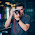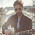VHDL coding tips and tricks: 8 bit Binary to BCD converter - Double Dabble algorithm

## Monday, April 19, 2010

### 8 bit Binary to BCD converter - Double Dabble algorithm

I have written a function for converting a 8-bit binary signal into a 12 bit BCD ( consisting of 3 BCD digits).An algorithm known as "double dabble" is used for this.The explanation for the algorithm can be found here.

The function code is given below:

function to_bcd ( bin : std_logic_vector(7 downto 0) ) return std_logic_vector is
variable i : integer:=0;
variable bcd : std_logic_vector(11 downto 0) := (others => '0');
variable bint : std_logic_vector(7 downto 0) := bin;

begin
for i in 0 to 7 loop  -- repeating 8 times.
bcd(11 downto 1) := bcd(10 downto 0);  --shifting the bits.
bcd(0) := bint(7);
bint(7 downto 1) := bint(6 downto 0);
bint(0) :='0';

if(i < 7 and bcd(3 downto 0) > "0100") then --add 3 if BCD digit is greater than 4.
bcd(3 downto 0) := bcd(3 downto 0) + "0011";
end if;

if(i < 7 and bcd(7 downto 4) > "0100") then --add 3 if BCD digit is greater than 4.
bcd(7 downto 4) := bcd(7 downto 4) + "0011";
end if;

if(i < 7 and bcd(11 downto 8) > "0100") then  --add 3 if BCD digit is greater than 4.
bcd(11 downto 8) := bcd(11 downto 8) + "0011";
end if;

end loop;
return bcd;
end to_bcd;

Some sample inputs and the corresponding outputs are shown below:
bin = "01100011"   ,     output = "0000 1001 1001"  (99).
bin = "11111110"   ,     output = "0010 0101 0100"  (254).
bin = "10111011"   ,     output = "0001 1000 0111"  (187).

The code is synthesisable, and the cell usage statistics for Virtex-5 FPGA is shown below:

# BELS                             : 24
#      GND                         : 1
#      LUT3                        : 1
#      LUT4                        : 2
#      LUT5                        : 12
#      LUT6                        : 7
#      MUXF7                       : 1
# IO Buffers                       : 20
#      IBUF                        : 8
#      OBUF                        : 12

Note :- The code can be modified to convert any length binary number to BCD digits.This require very little change in the code.

#### 27 comments:

1.Hi,

is it possible to create these netlist-graphics from the command-line using xilinx tools?

2.@marvin2k : See this link from xilinx.
http://www.xilinx.com/itp/xilinx4/data/docs/xst/command_line9.html

3.@vipin: this is to synthesize a design? I meant something like the "RTL Viewer" mentioned in other posts (sorry, in fact I wanted to post in another blogentry of your blog...) to view the generated netlist.

4.There is a command like this in that link :
run -ifn watchvhd.vhd -ifmt VHDL -ofn watchvhd.ngc -ofmt NGC -p xcv50-bg256-6 -opt_mode Speed
-opt_level 1

This will generate the ngc file.You have to open this file with a xilinx ngc file viewer.

5.Yes, and my question was if one can invoke such a tool from the commandline... sorry for this much text...

6.I havent explored command line options till.I guess you should try whatever is given in that link.Also share your experience here.

7.could we make it for n bit??

8.@rourab : yes. you just have to understand the concept.you can make it for n bit. But the code will be more complicated.

9.function to_bcd ( bin : std_logic_vector((n-1) downto 0) ) return std_logic_vector is
variable i : integer:=0;
variable j : integer:=1;
variable bcd : std_logic_vector(((4*q)-1) downto 0) := (others => '0');
variable bint : std_logic_vector((n-1) downto 0) := bin;

begin
for i in 0 to n-1 loop -- repeating 8 times.
bcd(((4*q)-1) downto 1) := bcd(((4*q)-2) downto 0); --shifting the bits.
bcd(0) := bint(n-1);
bint((n-1) downto 1) := bint((n-2) downto 0);
bint(0) :='0';

l1: for j in 1 to q loop
if(i < n-1 and bcd(((4*q)-1) downto ((4*q)-4)) > "0100") then --add 3 if BCD digit is greater than 4.
bcd(((4*q)-1) downto ((4*q)-4)) := bcd(((4*q)-1) downto ((4*q)-4)) + "0011";

end if;

end loop l1;
end loop;
return bcd;
end to_bcd;

10.the previous code i have written in generic form ,where q is the number bcd digit,in that case i got the desire result up to 9 but when it exceed over 9 it gives the A,B,C,D,E,F. i cant get my mistake,

11.vipin i have solved my problem just replacing the q by j in the inner loop

12.rourab, please, can you tell me which q you replaced by j in the inner loop?

I wrote similar code, but without generic parameter, and I had same problem (A,B,C..F).

13.function to_bcd ( bin : std_logic_vector((n-1) downto 0) ) return std_logic_vector is
variable i : integer:=0;
variable j : integer:=1;
variable bcd : std_logic_vector(((4*q)-1) downto 0) := (others => '0');
variable bint : std_logic_vector((n-1) downto 0) := bin;

begin
for i in 0 to n-1 loop -- repeating 8 times.
bcd(((4*q)-1) downto 1) := bcd(((4*q)-2) downto 0); --shifting the bits.
bcd(0) := bint(n-1);
bint((n-1) downto 1) := bint((n-2) downto 0);
bint(0) :='0';

l1: for j in 1 to q loop
if(i < n-1 and bcd(((4*j)-1) downto ((4*j)-4)) > "0100") then --add 3 if BCD digit is greater than 4.
bcd(((4*j)-1) downto ((4*j)-4)) := bcd(((4*j)-1) downto ((4*j)-4)) + "0011";

end if;

end loop l1;
end loop;
return bcd;
end to_bcd;

this is my code

14.rourab,

thank you very much, but I still have problem with this code, even when its generic.

I wanted to convert 24-bit binary to 32-bit bcd and I inserted your function into my code, where I defined q := 8, because (4*q)-1) would be 32 bits.

When I wanna do 8-bit bin to 12-bit bcd, I need to define q := 3.

My question is:

What is relation between n and q, what is relation between number of bits for input (bin) and number of bits for output (bcd)?

Please, answer again...I am beginner and your little help is very great for me.

Sory for my bad english, i hope that you can understand me.

15.I found answer for my question, but I need help for implementing that solution.

Relation between n and q is :

**********************************************
q = round(n*(log(2)))

where q must be rounded to greater integer !!!,
**********************************************

for example:

for n = 24 and q = 8 it would be:

q = round (32*(log (2)))= round (24*0.3010)=round (7.224)= 8

I need help for implement this calculus for generic parameter (I want to make automatic calculus q = f(n)).

Is this possible to be done with "real" data type,could we use not-synthesizable data type for generic parameter , to make synthesizable entity ?

16.how to implement for D-ALGORITHM IN TESTING OF VLSI CIRCUITS" IN VHDL OR VERILOG?"

17.Thanks, Men! That function helped me a lot. I made a physical implementation in a Spartan 3E using 8 switches as input and 3 displays as output. I wrote a post (Spanish) about it. I linked yours, of course!

18.Hi, I have the same question/problem which Aleksander mentioned above.

------------------------
Is this possible to be done with "real" data type,could we use not-synthesizable data type for generic parameter , to make synthesizable entity ?
------------------------
but, with a simpler implementation(from wiki): q = Roundup(n/3)

Could anyone help me with their views please ??
Thanks in advance.

19.Hello mate, I've written follow as your code above and my code is
LIBRARY ieee;
USE ieee.std_logic_1164.all;
use IEEE.std_logic_arith.all;
use IEEE.numeric_bit.all;
use IEEE.numeric_std.all;
use IEEE.std_logic_signed.all;

ENTITY ten_to_bcd IS
PORT ( b: IN std_logic_vector (7 downto 0) ; -- 10 bits input
y: OUT std_logic_vector( 11 downto 0)); -- 16 bits output / 4 digits
END ten_to_bcd;

ARCHITECTURE double_d OF ten_to_bcd IS

function dd( bin : std_logic_vector(7 downto 0) ) return std_logic_vector is
variable i : integer:=0;
variable bcd : std_logic_vector(11 downto 0) := "000000000000";
variable bint : std_logic_vector(7 downto 0) := bin;
variable kk : integer

begin
for i in 0 to 7 loop -- repeating 8 times.
bcd(11 downto 1) := bcd(10 downto 0); --shifting the bits.
bcd(0) := bint(7);
bint(7 downto 1) := bint(6 downto 0);
bint(0) :='0';

if(i < 7 and bcd(3 downto 0) > "0100") then --add 3 if BCD digit is greater than 4.
bcd(3 downto 0) := bcd(3 downto 0) + "0011";
end if;

if(i < 7 and bcd(7 downto 4) > "0100") then --add 3 if BCD digit is greater than 4.
bcd(7 downto 4) := bcd(7 downto 4) + "0011";
end if;

if(i < 7 and bcd(11 downto 8) > "0100") then --add 3 if BCD digit is greater than 4.
bcd(11 downto 8) := bcd(11 downto 8) + "0011";
end if;

end loop;
return bcd;
end dd;

begin
p:process(b)
begin
y <= dd(b);
end process;
END double_d;

so, I have some problem like when I run the simulation and when it get thought 15 , the value doesn't change to 16 but it shows 10 as tbl file mentioned below

0.0> 000 = 0000
10.0> 001 = 0001
20.0> 002 = 0002
30.0> 003 = 0003
40.0> 004 = 0004
50.0> 005 = 0005
60.0> 006 = 0006
70.0> 007 = 0007
80.0> 008 = 0008
90.0> 009 = 0009
100.0> 00A = 0010
110.0> 00B = 0011
120.0> 00C = 0012
130.0> 00D = 0013
140.0> 00E = 0014
150.0> 00F = 0015
160.0> 010 = 0010
170.0> 011 = 0011
180.0> 012 = 0012
190.0> 013 = 0013
200.0> 014 = 0020

could you please figure out what wrong with my code T^T
Thanksssss

1.Hi, did you managed to figure it out?
I am having the same issue.

Thanks!

20.This is my generic implementation:

function ToBcd(bin : word; nbrDecs : positive) return word is
variable bcd : word(4*nbrDecs-1 downto 0);
variable bint : word(bin'length-1 downto 0);
variable i, j : integer;
begin
bint := bin;
bcd := (others => '0');

for i in 0 to bin'high loop
bcd(bcd'high downto 1) := bcd(bcd'high-1 downto 0); -- Shift
bcd(0) := bint(bin'high);
--
bint(bint'high downto 1) := bint(bint'high-1 downto 0); -- Shift
bint(0) := '0';

for j in 0 to nbrDecs-1 loop
if (i < bin'high and bcd((j+1)*4-1 downto j*4) > "0100") then
bcd((j+1)*4-1 downto j*4) := bcd((j+1)*4-1 downto j*4) + "0011";
end if;
end loop;
end loop;
return bcd;
end function;

21.Working on a project that will be using a modified code for binary to dual bcd outs.

Having some issued mapping the inputs to our variables.

For example on input is marked as a and will be the MSB coming to the chipset.

There have been attempts to work it up as vin(7)<= a;

Would anyone be able to assist with explaining/helping/providing knowledge?

Thank you,

22.The main problem with your codes, is that when : '' add 3 if BCD digit is greater than 4 '' you do not pay attention of the eventual reminder, indeed if you have 1110, you add 3 because it is greater than 4, it should give you 10001 but you just keep 0001.
Sincerly,

1.What's the proper way to do this? I am getting the same result as Sahacha Nilkumhang.

23.does the same rule of this algorithm work with 16 bit values?

1.yes. it will work. its a generic algorithm. thought you have to rewrite the above code.

24.Hi guys,

Could you provide a testbench for this design?
Thank you very much.